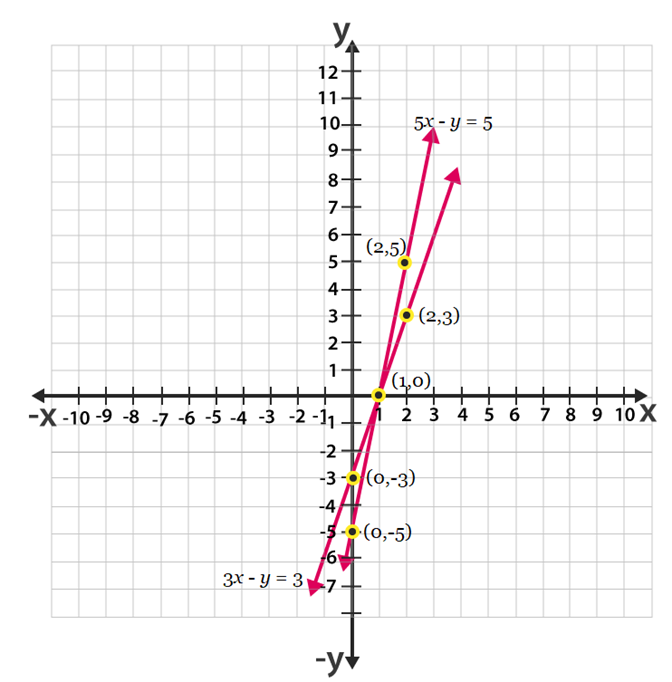# Draw the graphs of the equations 5x - y = 5 and 3x - y = 3. Determine the co-ordinates of the vertices of the triangle formed by these lines and the y axis.

Given equations

5x – y = 5—(i)

3x – y = 3—(ii)

From equation (i)

y = 5x – 5

From equation (ii)

y = 3x – 3

The solution table for 5x – y = 5

 x 2 1 0 y 5 0 -5

The solution table for 3x-y=3

 x 2 1 0 y 5 0 -5

The graphical representation of these lines will beThe above graph shows that the triangle formed is ∆ABC by the lines and the y axis. The coordinates of the vertices are A(1,0) , C(0,-5) and B(0,-3).